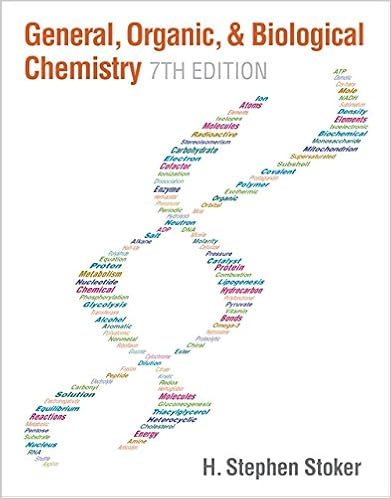1 v 2 2 cr 2 3 fe 3 4 co 2 5 ni 2 43 which of these

• Notes
• palmera
• 52
• 50% (2) 1 out of 2 people found this document helpful

This preview shows page 12 - 19 out of 52 pages.

We have textbook solutions for you!
The document you are viewing contains questions related to this textbook.The document you are viewing contains questions related to this textbook.
Chapter 16 / Exercise 16-49
General, Organic, & Biological Chemistry
StokerExpert Verified
(1) V 2+ (2) Cr 2+ (3) Fe 3+ (4) Co 2+ (5) Ni 2+
We have textbook solutions for you!
The document you are viewing contains questions related to this textbook.The document you are viewing contains questions related to this textbook.
Chapter 16 / Exercise 16-49
General, Organic, & Biological Chemistry
StokerExpert Verified
43. Which of these gas phase ions has the smallest number of unpaired electrons? Fe 3+ 7. Rank in the order of increasing ionic radius: Br , Te 2 , I
(1) Br < I < Te 2 (2) Br < Te 2 < I (3) I < Te 2 < Br (4) Te 2 < I < Br (5) Te 2 - < Br < I Ionic/Atomic Radius 30. Which is the largest: Ar, K + , Cl - , S 2- , or Ca 2+ ? 2+
Atomic Radius 8. Rank in order of increasing atomic radius: O, P, S (1) O < P < S (2) O < S < P (3) P < O < S (4) P < S < O (5) S < P < O 9. Arrange the following elements in order of increasing atomic radii. Sr, Rb, Sb, I, In (1) Rb < Sr < In < Sb< I (2) I < Sb < In < Rb < Sr (3) In < Sb < I < Sr < Rb (4) Sb < I < In < Sr < Rb (5) I < Sb < In < Sr < Rb 17. Which of the following elements has the largest atomic size? (5) Rn
Electron Config 10. Which of the following ground state electron configurations is incorrect? (1) Ca 1s 2 2s 2 2p 6 3s 2 3p 6 4s 2 (2) Mn [Ar] 4s (3) Cu [Ar] 3d 10 4s 1 (4) Sn [Kr] 4d 10 5s 2 (5) Xe [Kr] 4d 10 5s 2 5p 2 4d 5 5p 2 6
14. Select the correct electron configuration for Te (Z=52): 6 4
21. The ground state of which species has two half filled subshells?
Ionization Energy Trend 11. Arrange the following elements in order of decreasing first ionization energy. F, Be, B, N, C (1) F > B > N > C > Be (2) Be > C > B > N > F (3) F > N > B > C > Be (4) B > F > N > Be > C (5) Be > C > N > B > F Resonance 10. Which species requires the drawing of resonance structures? 2
54. Which of the following requires a resonance hybrid for its Lewis structure? (1) CO 2 (2) OCl - (3) CO 3 2- (4) OH - (5) H 2 O
46. How many important resonance structures may be drawn for PO 4 3− ? (4) 4
For which of these species is the best description of the bonding provided by two or more equivalent resonance structures?
11. All of the following about resonance are true except
Hybridization 18. A molecule in which sp 2 hybrid orbitals are employed for bond formation by the central atom is: (1) CO (2) SCl 2 (3) NH 3 (4) C 2 H 2 (5) Bond Length H 2 CO 12. Which of the following species has the shortest bond length?
O 2 19. The electronic transitions responsible for the Balmer lines in the H
atom terminate on n=2. What is the n value for the upper level if the wavelength emitted is 434 nm?
•••